# Kutta-Merson method

(diff) ← Older revision | Latest revision (diff) | Newer revision → (diff)

A five-stage Runge–Kutta method with fourth-order accuracy. Applied to the Cauchy problem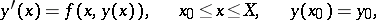(1)

the method is as follows:(2)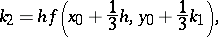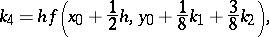The numberserves as an estimate of the error and is used for automatic selection of the integration step. Ifis the prescribed accuracy of the computation, the integration step is selected as follows. First choose some initial step and start the computation by (2) to obtain the number. If, divide the integration step by 2; if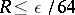, double it. If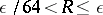, the selected integration step is satisfactory. Now replace the initial pointby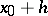and repeat the entire procedure. This yields an approximate solution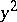; the quantity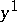is mainly auxiliary.

Since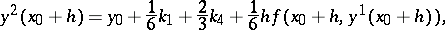i.e. the formula foris as it were "nested" in the formula for, the method described here for the estimation of the error and the selection of the integration step is known as an imbedded Runge–Kutta method.

Standard programs for the Kutta–Merson method are available in Algol , .